# Logistic Regression with a Neural Network mindset

yelim421·2021년 8월 17일
0

## Machine_Learning

목록 보기
2/24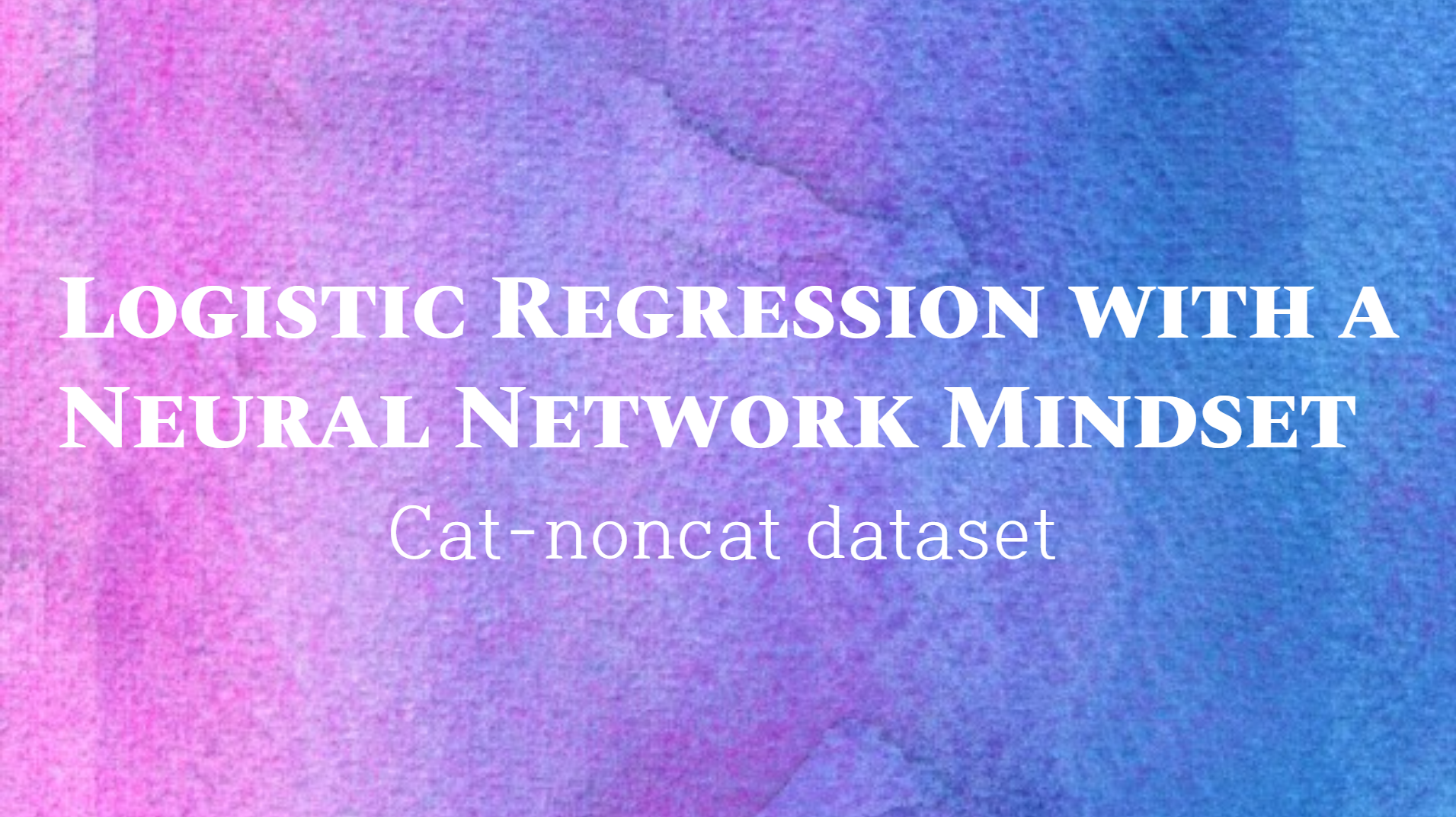#### Packages

import numpy as np
import copy
import matplotlib.pyplot as plt
import h5py
import scipy
from PIL import Image
from scipy import ndimage
from public_tests import *

%matplotlib inline
%autoreload 2

## Overview Problem set

아래서 사용하게 될 "data.h5" 데이터셋은

• cat (y=1)과 non-cat (y=0)으로 레이블된 m_train 트레인셋
• cat (y=1)과 non-cat (y=0)으로 레이블된 m_test 테스트셋
• 각각의 이미지는 (num_px, num_px, 3)의 shape을 가진다.

train_set_x_orig, train_set_y, test_set_x_orig, test_set_y, classes = load_dataset()

### Example of a picture

index = 25
plt.imshow(train_set_x_orig[index])
print ("y = " + str(train_set_y[:, index]) + ", it's a '" + classes[np.squeeze(train_set_y[:, index])].decode("utf-8") +  "' picture.")

output: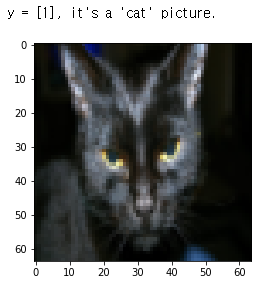### find the value of <m_train, m_test, num_px>

m_train = train_set_x_orig.shape
m_test = test_set_x_orig.shape
num_px = train_set_x_orig.shape

print ("Number of training examples: m_train = " + str(m_train))
print ("Number of testing examples: m_test = " + str(m_test))
print ("Height/Width of each image: num_px = " + str(num_px))
print ("Each image is of size: (" + str(num_px) + ", " + str(num_px) + ", 3)")
print ("train_set_x shape: " + str(train_set_x_orig.shape))
print ("train_set_y shape: " + str(train_set_y.shape))
print ("test_set_x shape: " + str(test_set_x_orig.shape))
print ("test_set_y shape: " + str(test_set_y.shape))

Number of training examples: m_train = 209
Number of testing examples: m_test = 50
Height/Width of each image: num_px = 64
Each image is of size: (64, 64, 3)
train_set_x shape: (209, 64, 64, 3)
train_set_y shape: (1, 209)
test_set_x shape: (50, 64, 64, 3)
test_set_y shape: (1, 50)

### Reshape dataset to flatten image of size(num_px, num_px, 3)->(num_px*num_px**3, 1)

train_set_x_flatten = train_set_x_orig.reshape(train_set_x_orig.shape,-1).T
test_set_x_flatten = test_set_x_orig.reshape(test_set_x_orig.shape,-1).T

train_set_x_flatten shape: (12288, 209)
train_set_y shape: (1, 209)
test_set_x_flatten shape: (12288, 50)
test_set_y shape: (1, 50)

### Standardize dataset

train_set_x = train_set_x_flatten / 255.
test_set_x = test_set_x_flatten / 255.

### 보편적인 프로세싱 순서:

1. 차원과 모양을 알아낸다
2. Reshape
3. Standardize

## General Architecture of the learning algorithm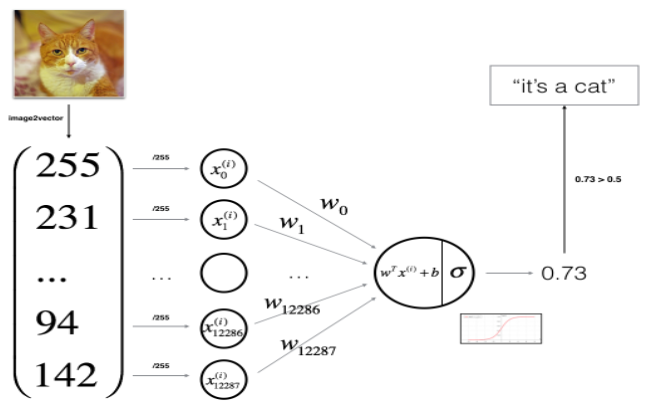1. Initialize parameters
2. Learn model by minimizing the cost
3. Make predictions (on test set)
4. Analyse result

### Sigmoid Function

def sigmoid(z):
s = 1/(1+np.exp(-z))
return s

### Initialize Parameters

def initialize_with_zeros(dim):
w = np.zeros([dim,1])
b = 0.
return w,b

dim = 2
w,b = initialize_with_zeros(dim)

### Forward and Backward propagation(Compute Cost function)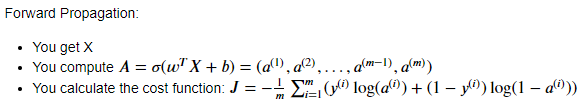use: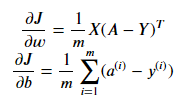input:
w - 가중치, array of size(num_px num_px **3, 1)
b - bias
X - 데이터 사이즈 (num_px
num_px **3, example 개수)
Y - "label" vector of size(1, example 개수)

.shape 이나 .shape이 헷갈린다면 여기를 참고하자.

def propagate(w, b, X, Y):
#forward propagation
m = X.shape
A = sigmoid(np.dot(w.T, X) + b)
cost = -1/m * (np.dot(Y, np.log(A).T) + np.dot((1-Y), np.log(1-A).T))

#backward propagation
dw = 1/m * (np.dot(X, (A-Y).T))
db = 1/m * (np.sum(A-Y))

cost = np.squeeze(np.array(cost))

return grads, cost

### Optimization (update parameters)

learn w & b by minimizing the cost function

#### update rule :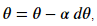where a is learning rate

def optimize(w, b, X, Y, num_iterations=100, learning_rate=0.009, print_cost=False):
w = copy.deepcopy(w)
b = copy.deepcopy(b)

costs=[]

for i in range(num_iterations):
grads, cost = propagate(w, b, X, Y)

w = w - learning_rate*dw
b = b - learning_rate*db

#Record costs
if i%100 ==0:
costs.append(cost)
if print_cost:
print(cost)

params = {"w":w, "b":b}

params, grads, costs = optimize(w, b, X, Y, num_iterations=100, learning_rate=0.009, print_cost=False)

print ("w = " + str(params["w"]))
print ("b = " + str(params["b"]))
print ("dw = " + str(grads["dw"]))
print ("db = " + str(grads["db"]))
print("Costs = " + str(costs))

### Predict

calculate: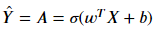convert the entries(data) into 0 or 1

def predict(w, b, X):
m = X.shape #한 샘플의 차원
Y_prediction = np.zeros((1, m))
w = w.reshape(X.shape, 1)

A = sigmoid(np.dot(x.T, X)+b)

for i in range(A.shape):
if (A[0,i]>0.5):
Y_prediction[0,i] = 1
else:
Y_prediction[0,i] = 0
return Y_prediction

## Merge All Functions into a model

def model(X_train, Y_train, X_test, Y_test, num_iterations=2000, learning_rate=0.5, print_cost=false):
w,b = initialize_with_zeros(X_train.shape)

parameters, grads, costs = optimize(w, b, X_train, num_iterations, learning_rate, print_cost)

w = parameters["w"]
b = parameters["b"]

Y_prediction_test = predict(w,b,X_test)
Y_prediction_train = predict(w,b,X_train)

## Analysis

# Plot learning curve (with costs)
costs = np.squeeze(logistic_regression_model['costs'])
plt.plot(costs)
plt.ylabel('cost')
plt.xlabel('iterations (per hundreds)')
plt.title("Learning rate =" + str(logistic_regression_model["learning_rate"]))
plt.show()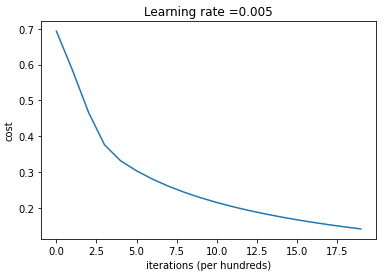• cost 는 줄어들고 있음(parameters are being learned)
• iterations을 높이면 training set에 대한 정확도는 높아질 수 있지만 test set에 대한 정확도는 낮아진다. => overfitting

### Learning Rate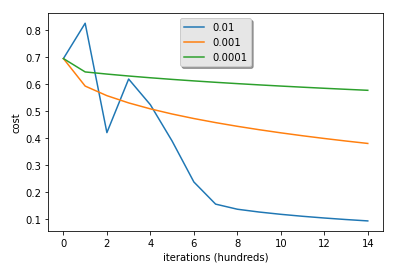• lr이 너무 크면 그래프가 왔다갔다 할 수 있음.
• lower cost가 더 좋은 모델이 아닐수도 있음. overfitting 인지 아닌지 확인해야 함. (overfitting은 train acc가 test보다 훨씬 클때 가능성이 있음)